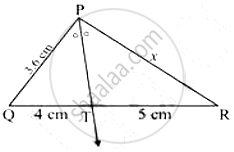# In the Following Figure, Ray Pt is the Bisector of ∠Qpr Find the Value of X and Perimeter of ∠Qpr. - Geometry

Sum

In the following figure, ray PT is the bisector of QPR Find the value of x and perimeter of QPR.#### Solution

In Δ PQR, PT is the bisector of P

"QT"/"TR" = "PQ"/"PR"

4/5 = 3.6/"PR"

4PR = 18

PR = 18/4= 9/2 = 4.5cm

QR =QT +TR

QR = 4+5 = 9 cm

Perimeter of Δ PQR =PQ+QR +PR

3.6+9+4.5 = 17.1 cm

Perimeter of Δ PQR = 17.1 cm

Concept: Property of an Angle Bisector of a Triangle
Is there an error in this question or solution?